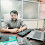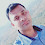# Set in Python

49
Set is a collection of finite elements, It does not contain any sequence(index) and it displays elements randomly.
Set displays unique elements and it will be declared using    {}  and Set().
Syntax of Set:-
var = {item1,item2, item3, ...}
var = set();
Example:-
x = {"C","CPP","DS","PHP","Java",".NET"}
or
x = set({"C","CPP","DS","PHP","Java",".NET"})
Example of Set:-
x = {"C","CPP","DS","PHP","Java",".NET","C","CPP"}
for data in x:
print(data)
How to add elements in Set from User Input?
Note:- Python use {} to declare dictionary and set both but {} is especially for dictionary hence to declare an empty set we should use set().
x = set()
size = int(input("enter size of set"))
for i in range(0,size):
item = input("Enter item")
print("Set elements is ")
for i in x:
print(i)
Where we use to set in python:-
1) When we want to display random elements then we can use set
2)  When we want to display unique elements then we can use set
for example, OTP is a random number it can be implemented by Set.
Predefined Method Of Set:-
x = {"C","CPP","DS","PHP","Java",".NET","C","CPP"}
2)  remove():-  It is used to remove an element in the set
x.remove("C")
3)  max(),min():-  it return max and minimum element
print(max(x))
print(min(x))
4) sum():-    It is used to calculate the sum of set elements
print(sum(x))
5)   union():-   It is used to combine two different subsets in a set.
a = {1,2,3,4}
b =  {2,3,7,8,9}
c= a.union(b)
6)  intersection():- It will return common elements from set
a = {1,2,3,4}
b =  {2,3,7,8,9}
c= a.intersection(b)
7) difference():-   It is used to use minus operation in the set means the elements which will exist under x will be removed from y.
a = {1,2,3,4}
b =  {2,3,7,8,9}
c= a.difference(b)
Complete Example of Set Using Program:-
x = {1,12,23,34,45,11,3}
y = {2,3}
x.remove(1)
print(max(x))
print(min(x))
print(sum(x))
print(x.union(y))
print(y.union(x))
print(x.intersection(y))
print(x.difference(y))
print("data is ")
for data in x:
print(data)
ASSIGNMENT of SET:-
1)  WAP to find the max element in a set not using max()?
2)  WAP to reverse set elements (if random then display actual random and reverse of random elements)?
s={3,11,23,34,56,1,78,19,17,98,1}
s1 = [int]*len(s)
c=0
for i in s:
s1[c]=i
c=c+1
for i in range(len(s)-1,-1,-1):
print(s1[i])
3)  WAP to display prime element in Set?
What is Frozenset?
This is the special set in python that can not be modified. means it is an immutable set. means we can not add and remove elements.
a = frozenset({1,2,3})
for d in a:
print(d)
Tags

1.#WAP to find the max element in a set not using max()?
m=0
s=set()
item=int(input("enter value that you want to insert"))
for i in range(0,item):
value=input("enter item")
for x in s:
if x>m:
m=x
print("max value is=",m)

2.#WAP to display prime element in Set?
s=set()
c=0
item=int(input("enter value want to check prime number"))

for i in range(0,item):
item=input("enter value")
for i in s:

if i%2==0:
c=c+1
break
if c==0:
print("This is a prime number",i)
else:
print('This is a not number.')

1.this is wrong program

3.Q:- WAP to find the max element in a set not using max() ??
Solution:-
a=0
s=set()
item=int(input("enter value that you want to insert"))
for i in range(0,item):
value=input("enter item")
for b in s:
if b>a:
a=b
print("max value is=",a)

4.Q:- WAP to display prime element in Set ??
Solution:-
a=set()
x=0
item=int(input("enter value want to check prime number"))
for i in range(0,item):
item=input("enter value")
for i in a:
if i%2==0:
x=x+1
break
if x==0:
print("This is a prime number",i)
else:
print('This is a not number.')

5.DOUBT------

Program to find max in set-->>

x=set()
h=0
size=int(input("enter size of set"))
for i in range(0,size):
element=input("enter element")
if x>h:
h=x
print("max is",h)

Error-->>

if x>h:
TypeError: '>' not supported between instances of 'set' and 'int'

Sir kaise execute kare????

6.x=set()
h=0
size=int(input("enter size of set"))
for i in range(0,size):
element=input("enter element")

for x1 in x:
if x1>h:
h=x1
print("max is",h)

7.DOUBT----->Program of max element in set-->

x=set()
h=0
size=int(input("enter size of set"))
for i in range(0,size):
element=input("enter element")

for x1 in x:
if x1>h:
h=x1
print("max is",h)

Error-->

if x1>h:
TypeError: '>' not supported between instances of 'str' and 'int'

8.DOUBT-->>Program to display prime number in a set-->>

x=set()
p=0
size=int(input("enter the size of set"))
for i in range(0,size):
num=input("enter the number")
for i in x:
if i%2==0:
p=p+1
if p==2:
print("it is prime number")
else:
print("it is not a prime number")

ERROR-->>
if i%2==0:
TypeError: not all arguments converted during string formatting

9.deependra singh jadaunNovember 27, 2020 at 6:12 PM

wap tofind max. element from given no. of set elements:
x={1,2,3,4,5,6,7,8,9,56,50,0}
m=0
for i in x:
if i>m:
m=i
print(m)

10.deependra singh jadaunNovember 27, 2020 at 11:23 PM

wap to display prime elements from a given set:

x={2,3,4,5,6,7,8,9,10}
for j in x:

for i in range (2,j):
if j%i ==0:
print("this number is not prime ", j)
break
else:
print("this is prime element", j)

1.x={2,3,4,5,6,7,8,9,10}
for j in x:
for i in range (2,j):
if j%i ==0:
break
else:
print(j)

2.x={2,3,4,5,6,7,8,9,10}
y=set()
for j in x:
for i in range (2,j):
if j%i ==0:
break
else:

print(y)

11.#Max element in set
a=0
x=set()
s= int(input('How many numbers in set = '))

for j in range(0,s):
num = int(input("Enter number = "))
print(x)
for i in x:
if i>a:
a=i

print("Max number is = ",a)

12.# Python program to find largest
# number in a list

# list of numbers
list1 = [10, 20, 4, 45, 99]

# sorting the list
list1.sort()

# printing the last element
print("Largest element is:", list1[-1])

13.s={3,11,23,34,56,1,78,19,17,98,1}

s1 = [int]*len(s)
c=0
for i in s:
s1[c]=i
c=c+1
for i in range(len(s)-1,-1,-1):
print(s1[i])

14.#WAP to display prime element in Set?
a = {3,4,5,6,7,8,9}

for i in a:
for j in range(2,i):
if i%j == 0:

break
else :
k=i
print(k,"is a Prime no.")

15.# PYTHON (6 To 7 PM BATCH)
# CODE to find the Max Element in a Set not using Max().

a = {1,2,3,11,7,4,5,6}
d = 0

for c in a:
if c>d:
d = c

print(c)

16.# PYTHON ( 6 To 7 PM BATCH)
# CODE to Reverse Set Elements,

s={3,11,23,34,56,1,78,19,17,98,1}
s1 = []
z = []

for i in s:
s1.append(i)

for y in range(len(s1)-1,-1,-1):
z.append(s1[y])

a = set(z)
print("Reverse of a set Elements:-",a)

17.# PYTHON ( 6 To 7 PM BATCH)

# WAP to display prime element in Set

s = {3, 11, 23, 34, 56, 1, 78, 19, 17, 98, 1}

for y in s:
z = 0
for i in range(1,y+1):
if y%i == 0:
z += 1

if z==2 or z==1:
print("Prime :-",y)
else:
print("Not Prime:-",y)

18.#Akash patel
wap to find max. element from given no. of set elements:
x={1,2,3,4,5,6,7,8,9,10,15,20}
m=0
for data in x:
if data>m:
m=data
print(m)

19.# WAP TO display prime number in set?
num = {1,2,3,4,5,6,7,8,9,10,11,12,13,14,15}
primes = set()

for i in range(2, len(num)):
for j in range(2, int(i ** 0.5) + 1):
if i%j == 0:
break
else:

print(primes)

20.WAP to find the max element in a set not using max()

x={23,45,54,12,52,20,75}
max1=0
for i in x:
if max1<i:
max1=i
else:
continue
print(max1)

21.WAP to reverse set elements

x={23,45,54,12,52,20,75}
l=[]
for i in x:
l.append(i)
print("original list: ",l)
print("reverse list: ", l[::-1])

22.WAP to display prime element in Set

x={2,3,4,5,6,7,8,9,10}
for num in range(0,9):
if num>1:
for i in range(2,num):
if (num%i)==0:
break
else:
print(num)

23.x={1,25,56,5,854,62,5,65,84,65,18,4}
m=0
for i in x:
if m<i:
m=i
print(m)

24.s={2,69,52,62,85,45,18,94,51,61,88,456}
s1=[int]*len(s)
c=0
for i in s:
s1[c]=i
c=c+1

for i in range(len(s)-1,-1,-1):
print(s1[i])

25.s={2,69,52,62,85,45,18,94,51,61,88,456}
for n in s:
if n>1:
for i in range(2,n):
if(n%i)==0:
break
else:
print(n)

26.to find the max element in a set not using max()

m=0
s=set()
item=int(input("enter value that you want to insert"))
for i in range(0,item):
value=int(input("enter item"))
for x in s:
if x>m:
m=x
print("max value is=",m)

27.to display prime elements from a given set

s={2,3,4,5,6,7,8,9,10}
y=set()
for j in s:
for i in range (2,j):
if j%i ==0:
break
else:
print(" prime element is : ",y)

28.to reverse set elements

x={23,45,54,12,52,20,75}
l=[]
for i in x:
l.append(i)
print("Set element is : ",l)
print("reverse Set element is : ", l[::-1])

29.# WAP to find the max element in a set not using max()?
s=set()
l1=[]
m=0
l=int(input("enter the length of set:-"))
for i in range(0,l):
num=int(input("enter element:-"))
l1.append(num)
print("set is:-",s)
for i in range(0,len(l1)):
if l1[i]>m:
m=l1[i]
print("max is:-",m)

30.#WAP to reverse set elements (if random then display actual random and reverse of random elements)?
l=[]
s={12,34,56,67,78,5}
for i in s:
l.append(i)
print("set is:-",s)
print("reverse is:-")
for j in range(len(l)-1,-1,-1):
print(l[j])

31.#WAP to display prime element in Set?
l=[]
s={1,5,3,725,9,2,12}
for i in s:
l.append(i)
for j in range(0,len(l)):
for i in range(2,l[j]):
if l[i]%i==0:
break
else:
print(l[j])

32.#WAP to reverse set elements?

x={1,2,3,4}
print(x)
l=[]
for i in x:
l.append(i)
l.reverse()
print(l)

33.#WAP to find the max element in a set not using max()?
x={1,2,3,4,5,6,7,8,9}
max=0
for i in x:
if i>max:
max=i
print(max)

34.#Wap program to find max element in set?

st={20,4,54,90,51,55,80}
m=0
for i in st:
if m<i:
m=i
print(m)

35.x = {"C","CPP","DS","PHP","Java",".NET","C","CPP"}
print(x)

36.#WAP to display prime element in Set?
l=[1,13,5,3,9,2,7,12]
l1=[]
for i in l:
c=0
for j in range(1, i):
if(i% j == 0):
c=c+1
if c==1:
l1.append(i)
print(l1)

37.# dice game
import time
import random
dice=set([1,2,3,4,5,6])
hit1=0
hit2=0
score1=0
score2=0
print("Team 'A' is start Rolling")
flag=True
while flag:
d=random.choice(tuple(dice))
score1+=(d)
hit1+=1
if hit1==10:
flag=False
time.sleep(1)
print("Rolling no ",hit1,"& dice value ",d,"Till time Score ",score1)
print("Team 'B' is start Rolling")
flag=True
while flag:
d=random.choice(tuple(dice))
score2+=(d)
hit2+=1
if hit2==10:
flag=False
time.sleep(1)
print("Rolling no ",hit2,"& dice vlaue ",d,"Till time Score ",score2)
if score1>score2:
print("Winner is 'A' Team & score is "+str(score1))
else:
print("Winner is 'B' Team & score is "+str(score2))

38.# dice game
import time
import random
dice=set([1,2,3,4,5,6])
hit1=0
hit2=0
score1=0
score2=0
print("Team 'A' is start Rolling")
flag=True
while flag:
d=random.choice(tuple(dice))
score1+=(d)
hit1+=1
if hit1==10:
flag=False
time.sleep(1)
print("Rolling no ",hit1,"& dice value ",d,"Till time Score ",score1)
print("Team 'B' is start Rolling")
flag=True
while flag:
d=random.choice(tuple(dice))
score2+=(d)
hit2+=1
if hit2==10:
flag=False
time.sleep(1)
print("Rolling no ",hit2,"& dice vlaue ",d,"Till time Score ",score2)
if score1>score2:
print("RESULT --Winner is 'A' Team & score is "+str(score1))
elif score1==score2:
print("Match Draw :-'A' Team & score is "+str(score1)," 'B' Team & score is "+str(score2))

else:
print("RESULT --Winner is 'B' Team & score is "+str(score2))

39.#DICE GAME
import time
import random
d1=0
s1=0
d2=0
s2=0
dice1=[1,2,3,4,5,6,5,3,4,5,6,6,2,1]
dice2=[1,2,3,4,5,6,5,3,4,5,6,6,2,1]

for x in random.sample(dice1,9):
s1=s1+x
d1=d1+1
time.sleep(2)
if s1>20:
break
print(d1,"attempts","number is come by dice RAJ", x," total count score is>",s1)

for y in random.sample(dice2,9):
s2=s2+y
d2=d2+1
time.sleep(2)
if s2>20:
break
print(d2,"attempts","number is come by dice ABHISHEK", y," total count score is>",s2)
if d1==d2:
print("draw")
elif d1<d2:
print("raj win")
else:
print("abhishek win")

40.#WAP to display prime element in Set?
x=set()
size = int(input("enter the size of set"))
for i in range (0,size):
element = input("enter no.")

for j in x:
j=int(j)
if j%2!=0:
print(j)

41.#when we want to display unique elements then we can use set?
x={12,56,78,45,45,56,67,34,12,34}
y=set()
for i in x:
if i not in y:
print(y)

42.#when we want to display random elements then we can use set?
x={"apple","orange","banana","date"}
for i in x:
print(i)

43.#wap to display prime element in set?
s={2,3,4,6,8,7,9,11,53,15}
for num in s:
if num>1:
for i in range(2,num):
if num%i==0:
break
else:
print(num)

44.#wap to find max ele in a set not using max()?
s={67,90,30,50,200}
m=0
for i in s:
if m<i:
m=i
print("max value is",m)

45.WAP to find the max element in a set not using max()?

s=set()
print("enter 5 numbers for the set: ")
for i in range(0,5):
ele=int(input())
m=0
n=ele
for i in s:
if i>m:
m=i
if i<n:
n=i
print("The max num in the set is : ",m)
print("Also the min num is : ",n)

46.WAP to display prime element in Set?

s={1,2,3,4,5,6,7,8,9}
print("The set is: ",s)
print("The prime numbers in the above set are: ")
for i in s:
for j in range(2,i):
if i%j==0:
break
else:
print(i,end=" ")